Understanding Fixed-Income Risk and Return | IFT World
IFT Notes for Level I CFA® Program
IFT Notes for Level I CFA® Program

# Part 4

## 8. Money Duration and the Price Value of a Basis Point

The money duration of a bond is a measure of the price change in units of the currency in which the bond is denominated, given a change in annual yield to maturity.

Money Duration = AnnModDur x PVFULL

ΔPVFULL≈ -MoneyDur x Δyield

Consider a bond with a par value of $100 million. The current yield to maturity (YTM) is 5% and the full price is$102 per $100 par value. The annual modified duration of this bond is 3. the money duration can be calculated as the annual modified duration (3) multiplied by the full price ($102 million): 3 x $102 million =$306 million. If the YTM rises by 1% (100 bps) from 5% to 6% the decrease in value will be approximately $306 million x 1% =$3.06 million. If the YTM rises by 0.1% (10 bps), the decrease in value will be $306 million x 0.1% =$0.306 million.

An important measure which is related to money duration is the price value of a basis point (PVBP). The PVBP is an estimate of the change in the full price given a 1 bp change in the yield-to-maturity. The formal equation is given below.

PVBP =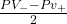where PV_ and PV+ are full prices calculated by decreasing and increasing the YTM by 1 basis point.

A quick way of calculating the price value of a basis point is to take the money duration and multiply by 0.0001. For example, if the money duration of a portfolio is $200,000 the price value of a basis point is$200,000 x 0.0001 = $20. (1 bp = 0.01% = 0.0001) Example 13: Calculating money duration of a bond A life insurance company holds a USD 1 million (par value) position in a bond that has a modified duration of 6.38. The full price of the bond is 102.32 per 100 of face value. 1. Calculate the money duration for the bond. 2. Using the money duration, estimate the loss for each 10 bps increase in the yield-to-maturity. Solution: 1. First calculate the full price of the bond:$1,000,000 x 102.32% = $1,023,200. The money duration for the bond is: 6.38 ×$1,023,200 = $6,528,000. 2. 10 bps corresponds to 0.10% = 0.0010. For each 10 bps increase in the yield-to-maturity, the loss is estimated to be:$6,528,000 × 0.0010 = $6,528.02. Example 14: Calculating PVBP for a bond Consider a$100, five-year bond that pays coupons at a rate of 10% semi-annually. The YTM is 10% and it is priced at par. The modified duration of the bond is 3.81. Calculate the PVBP for the bond.

Solution:

Money duration = $100 x 3.81 =$381.00

PVBP = $381 x 0.0001 =$0.0381

## 9. Bond Convexity

The graph below shows the relationship between bond price and YTM. It shows the convexity for a traditional fixed-rate bond.

Source: CFA Program Curriculum, Understanding Fixed-Income Risk and Return

Interpretation of the diagram:

• Duration assumes there is a linear relationship between the change in a bond’s price and change in YTM. For instance, assume the YTM of a bond is 10% and it is priced at par (100). According to the duration measure, if the YTM increases to 11% the price moves down to a point on the straight line.
• Similarly, the price moves up to a point on the straight line if the YTM decreases.
• The curved line in the above exhibit plots the actual bond prices against YTM. So in reality, the bond prices do not move along a straight line but exhibit a convex relationship.
• For small changes in YTM, the linear approximation is a good representation for change in bond price. That is, the difference between the straight and curved line is not significant.
• In other words, modified duration is a good measure of the price volatility.
• However, for large changes in YTM or when the rate volatility is high, a linear approximation is not accurate and a convexity adjustment is needed.

Here we need to factor in the convexity. The percentage change in the bond’s full price with convexity-adjustment is given by the following equation:

Change in the price of a full bond:

% ΔPVFULL =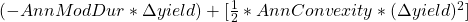Expression in first braces: duration adjustment

Expression in second braces: convexity adjustment

Approximate convexity can be calculated using this formula:

Approx. Convexity =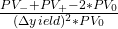where:

PV_ and PV+ = new full price when YTM is decreased and increased by the same amount

PV= original full price

The change in the full price of the bond in units of currency given a change in YTM can be calculated using this formula: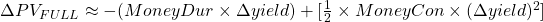Convexity is good

The following exhibit shows the price-yield curves for two bonds with the same YTM, price, and modified duration, and why greater convexity is good for an investor.

Interpretation of the diagram:

• Both the bonds have the same tangential line to their price-yield curves.
• When YTM decreases by the same amount, the more convex bond appreciates more in price.
• When YTM increases by the same amount, the more convex bond depreciates less in price than the less convex bond.
• The bond with greater convexity outperforms when interest rates go up/down.

The relationship between various bond parameters with convexity is the same as with duration.

For a fixed-rate bond,

• The lower the coupon rate, the greater the convexity.
• The lower the yield to maturity, the greater the convexity.
• The longer the time to maturity, the greater the convexity.
• The greater the dispersion of cash flow or cash payments spread over time, the greater the convexity.

Effective Convexity

For bonds whose cash flows were unpredictable, we used effective duration as a measure of interest rate risk. Similarly, we use effective convexity to measure the change in price for a change in benchmark yield curve for securities with uncertain cash flows. The effective convexity of a bond is a curve convexity statistic that measures the secondary effect of a change in a benchmark yield curve. It is used for bonds with embedded options.

Effective Convexity =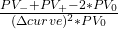Here is a summary of some important points related to bonds with embedded options:

• When the benchmark yield is high, prices of callable and non-callable bonds change almost similarly for interest rate changes.
• When the benchmark yield is low, call option becomes more valuable to the issuer.
• Callable bond exhibits negative convexity.
• Putable bonds always have positive convexity.

Example 15: Calculating the full price and convexity-adjusted percentage price change of a bond

A German bank holds a large position in a 6.50% annual coupon payment corporate bond that matures on 4 April 2029. The bond’s yield to maturity is 6.74% for settlement on 27 June 2014, stated as an effective annual rate. That settlement date is 83 days into the 360-day year using the 30/360 method of counting days.

1. Calculate the full price of the bond per 100 of par value.
2. Calculate the approximate modified duration and approximate convexity using a 1 bp increase and decrease in the yield to maturity.
3. Calculate the estimated convexity-adjusted percentage price change resulting from a 100 bp increase in the yield to maturity.
4. Compare the estimated percentage price change with the actual change, assuming the yield to maturity jumps to 7.74% on that settlement date.

Solution:

There are 15 years from the beginning of the current period on 4 April 2014 to maturity on 4 April 2029.

1. The full price of the bond is 99.2592 per 100 of par value.

FV = 100, I/Y = 6.74, PMT = 6.50, N = 15, CPT PV; PV = -97.777.

Full Price =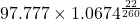= 99.2592.

2. PV+ = 99.1689

FV = 100, PMT = 6.5, I/Y = 6.75, N = 15, CPT PV; PV = -97.687.

PV+ =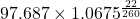= 99.1689.

PV_ = 99.3497.

FV = 100, I/Y = 6.73, PMT = 6.5, N = 15, CPT PV; PV = -97.869.

PV_ =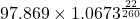= 99.3497.
ApproxModDur =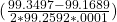= 9.1075.

The approximate modified duration is 9.1075.

ApproxCon =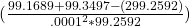= 201.493

The approximate convexity is 201.493.
3. The convexity-adjusted percentage price drop resulting from a 100 bp increase in the yield-to-maturity is estimated to be -8.1% (-9.1075 + 1.00746).<
Modified duration alone estimates the percentage drop to be 9.1075%. The convexity adjustment adds 100.746 bps (0.5 × 201.493 × .012 = 1.00746%).
4. The new full price if the yield-to-maturity goes from 6.74% to 7.74% on that settlement date is 90.7623. The actual percentage change in the bond price is -8.5603%. The convexity-adjusted estimate is -8.1%.
Example 16: Calculating the approximate modified duration and approximate convexity

The investment manager for a US defined-benefit pension scheme is considering two bonds about to be issued by a large life insurance company. The first is a 25-year, 5% semiannual coupon payment bond. The second is a 75-year, 5% semiannual coupon payment bond. Both bonds are expected to trade at par value at issuance.

Calculate the approximate modified duration and approximate convexity for each bond using a 5 bp increase and decrease in the annual yield-to-maturity.

Solution:

In the calculations, the yield per semiannual period goes up by 2.5 bps to 2.525% and down by 2.5 bps to 2.475%.

The 25-year bond has an approximate modified duration of 14.18.

PV+ : FV = 100, I/Y = 2.525, PMT = 2.5, N = 50, CPT PV, PV = -99.2945.

PV : FV = 100, I/Y = 2.475, PMT = 2.5, N = 50, CPT PV, PV = -100.7126.

ApproxModDur =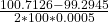= 14.18 and an approximate convexity of 284.
ApproxCon =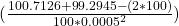= 284.

Similarly, the 75-year bond has an approximate modified duration of 19.51 and an approximate convexity of 708.